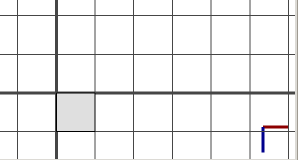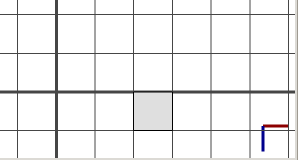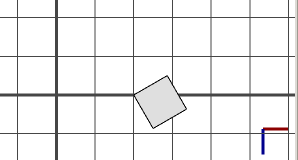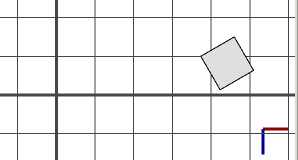# t operation

### Syntax

• t(tx, ty, tz)

### Parameters

1. txfloat
Amount to translate in the x direction.
2. tyfloat
Amount to translate in the y direction.
3. tzfloat
Amount to translate in the z direction.

### Description

The t operation translates the scope by the vector (tx, ty, tz), i.e. the vector is added to scope.t. If the scope rotation is non-zero, then the passed translation vector is rotated around the pivot first, with angles (scope.rx, scope.ry, scope.rz). In other words, the translation is relative to the scope axes.

The relative operator ' permits a convenient notation relative to the scope size: t('tx,0,0) is equivalent to t(tx*scope.sx, 0, 0).

Note: t(x,y,z) is the same as translate(rel, scope, x, y, z).

### Examples

#### Translate - rotation concatenation

 This is the initial shape we start with. ``````A--> primitiveCube()``````First a translation of two units along the x-axis. ``````A--> primitiveCube() t(2,0,0)``````Then a rotation of 30 degrees around the y-axis. ``````A--> primitiveCube() t(2,0,0) r(0,30,0)``````And another translation of 2 units along the x-axis. Translations are along the scope's x-axis, i.e. the rotation changes the global translation direction.The relative operator ' is used - here it does not make a difference because scope.sx is 1.``````A--> primitiveCube() t(2,0,0) r(0,30,0) t('2,0,0)``````